# Stoichiometry MoleMole Mole Ratio A mole ratio is

• Slides: 9Stoichiometry Mole-Mole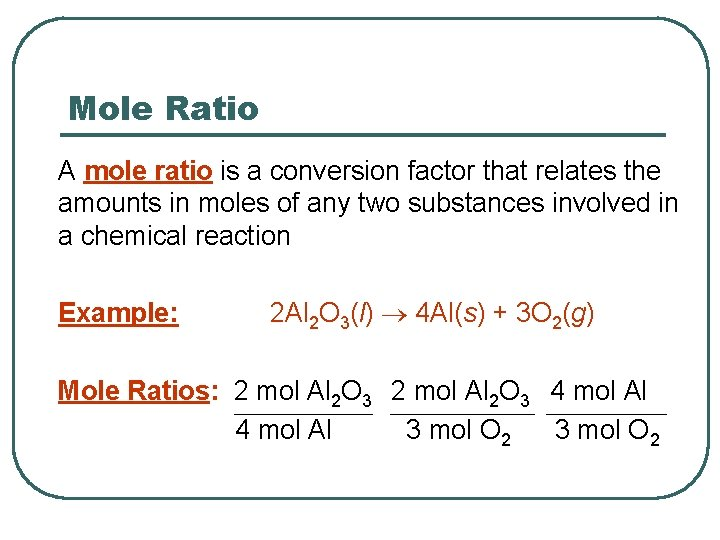Mole Ratio A mole ratio is a conversion factor that relates the amounts in moles of any two substances involved in a chemical reaction Example: 2 Al 2 O 3(l) 4 Al(s) + 3 O 2(g) Mole Ratios: 2 mol Al 2 O 3 4 mol Al 3 mol O 2Steps in Solving Problems… 1. 2. 3. 4. Write a balanced chemical equation Identify the given (known) and the unknown Write out your given, mole ratio (unknown/known) Multiply or divide to get your answer and don’t forget your units!!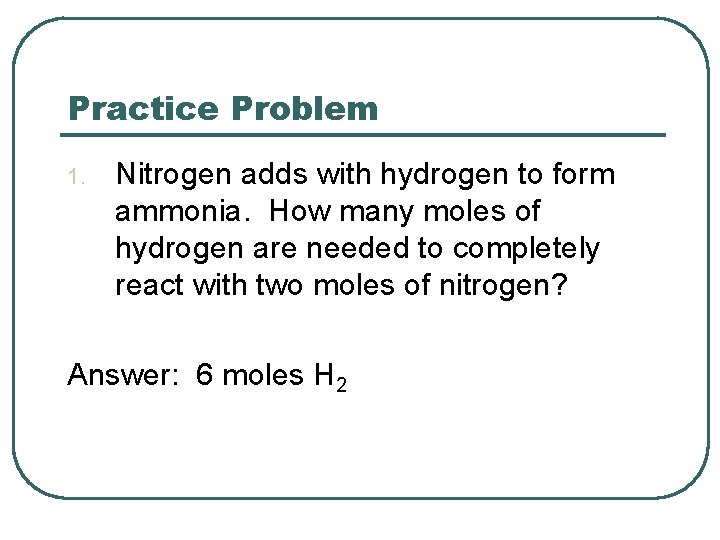Practice Problem 1. Nitrogen adds with hydrogen to form ammonia. How many moles of hydrogen are needed to completely react with two moles of nitrogen? Answer: 6 moles H 2Practice Problem 2. Potassium chlorate decomposes to form potassium chloride and oxygen gas. How many moles of oxygen are produced by the decomposition of six moles of potassium chlorate? Answer: 9 moles O 2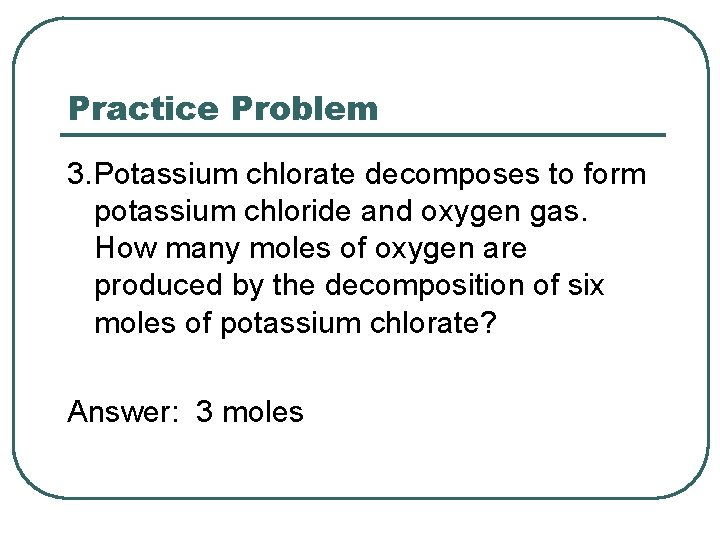Practice Problem 3. Potassium chlorate decomposes to form potassium chloride and oxygen gas. How many moles of oxygen are produced by the decomposition of six moles of potassium chlorate? Answer: 3 moles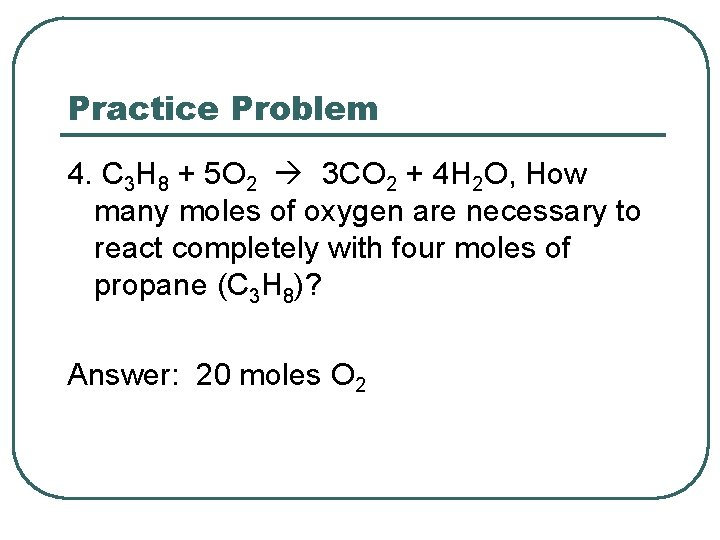Practice Problem 4. C 3 H 8 + 5 O 2 3 CO 2 + 4 H 2 O, How many moles of oxygen are necessary to react completely with four moles of propane (C 3 H 8)? Answer: 20 moles O 2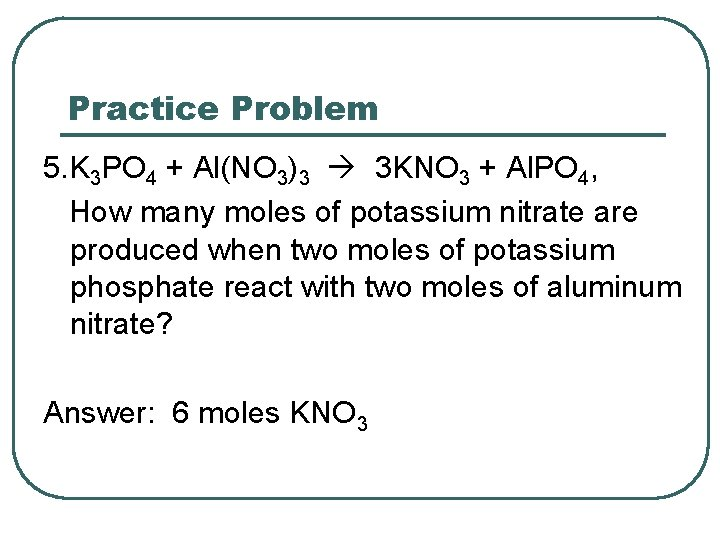Practice Problem 5. K 3 PO 4 + Al(NO 3)3 3 KNO 3 + Al. PO 4, How many moles of potassium nitrate are produced when two moles of potassium phosphate react with two moles of aluminum nitrate? Answer: 6 moles KNO 3